Levels of Measurement

Measurement of some attribute of a set of objects is the process of assigning numbers or other symbols to the objects in such a way that properties of the numbers or symbols reflect properties of the attribute being measured. There are different levels of measurement that involve different properties (relations and operations) of the numbers or symbols. Associated with each level of measurement is a set of transformations of the measurements that preserve the relevant properties; these transformations are called permissible transformations. A particular way of assigning numbers or symbols to measure something is called a scale of measurement.

The most commonly discussed levels of measurement are as follows:

Nominal

Two objects are assigned the same symbol if they have the same value of the attribute. Permissible transformations are any one-to-one or many-to-one transformation, although a many-to-one transformation loses information.

Ordinal

Objects are assigned numbers such that the order of the numbers reflects an order relation defined on the attribute. Two objects x and y with attribute values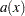and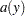are assigned numbers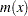and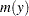such that if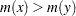, then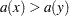. Permissible transformations are any monotone increasing transformation, although a transformation that is not strictly increasing loses information.

Interval

Objects are assigned numbers such that differences between the numbers reflect differences of the attribute. If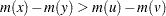, then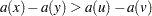. Permissible transformations are any affine transformation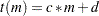, where c and d are constants; another way of saying this is that the origin and unit of measurement are arbitrary.

Log-interval

Objects are assigned numbers such that ratios between the numbers reflect ratios of the attribute. If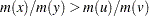, then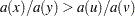. Permissible transformations are any power transformation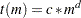, where c and d are constants.

Ratio

Objects are assigned numbers such that differences and ratios between the numbers reflect differences and ratios of the attribute. Permissible transformations are any linear (similarity) transformation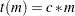, where c is a constant; another way of saying this is that the unit of measurement is arbitrary.

Absolute

Objects are assigned numbers such that all properties of the numbers reflect analogous properties of the attribute. The only permissible transformation is the identity transformation.

Proximity measures provided in the DISTANCE procedure accept four levels of measurement: nominal, ordinal, interval, and ratio. Ordinal variables are transformed to interval variables before processing. This is done by replacing the data with their rank scores, and by assuming that the classes of an ordinal variable are spaced equally along the interval scale. See the RANKSCORE= option in the section PROC DISTANCE Statement for choices on assigning scores to ordinal variables. There are also different approaches for how to transform an ordinal variable to an interval variable. See Anderberg (1973) for alternatives.